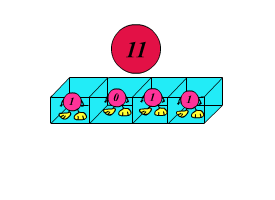Competitions

# Cycle shifts

Lets write the decimal integer n in binary notation and create all its left cycle shifts where first digit of the number goes to the end.

For example, if n = 11, than it will be 1011 in binary notation, cycle shifts are 0111, 1110, 1101, 1011. So the maximal value m among all received numbers equals to 11102 = 1410.

For the number n find the maximal value of m.#### Input

One number n (1n2 ·109).

#### Output

One number m.

Time limit 1 second
Memory limit 128 MiB
Input example #11
11

Output example #11
14